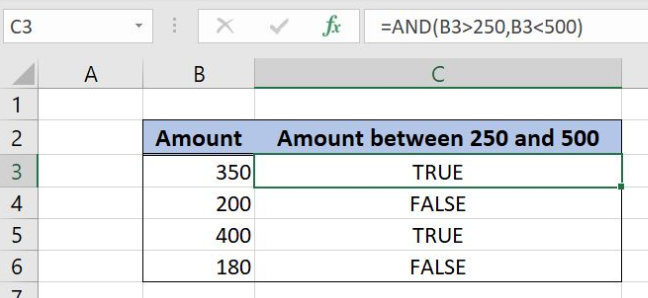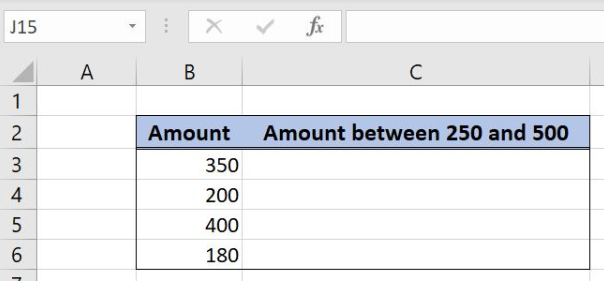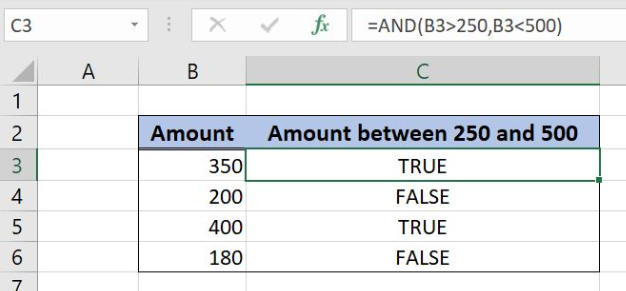Get instant live expert help with Excel or Google Sheets“My Excelchat expert helped me in less than 20 minutes, saving me what would have been 5 hours of work!”

#### Post your problem and you’ll get Expert help in seconds.

Your message must be at least 40 characters
Our professional Expert are available now. Your privacy is guaranteed.

# What Is the Excel AND Function and How You Can Use ItRead time: 25 minutes

Excel allows a user to use a logical expression in order to check if several conditions are met. This is possible by using the AND function which returns Boolean TRUE if all conditions are met, or FALSE if at least one condition is not met. This step by step tutorial will assist all levels of Excel users in using the AND function.Figure 1. The result of the AND function

## Syntax of the AND Formula

The generic formula for the AND function is:

`=AND(logical1, logical2, … )`

The parameters of the AND function are:

• logical1, logical2, … – logical expressions which need to be true.

## Setting up Our Data for the AND Formula

Let’s first look at the structure of the data. Our table has 2 columns: “Amount” (column B) and “Amount between 250 and 500” (column C). In column C we want to check if the value from column B is between 250 and 500 and return TRUE or FALSE.Figure 2. The data that we will use in the AND example

## Using the AND Function to Check Two Logical Expressions

In our example, we want to check if the value in B3 (350) is between 250 and 500 and get the result in C3. Therefore we need two have two logical expressions: The value needs to be greater than 250 and less than 500. If both conditions are true, the formula returns TRUE.

The formula looks like:

`=AND(B3>250, B3<500)`

The parameter logical1 is B3>250, and the logical2 is B3<500.

To apply the AND function, we need to follow these steps:

• Select cell C3 and click on it
• Insert the formula: `=AND(B3>250, B3<500)`
• Press enter
• Drag the formula down to the other cells in the column by clicking and dragging the little “+” icon at the bottom-right of the cell.Figure 3. Using the AND function

The B3 value, 350, is greater than 250 and also less than 500. Because both conditions are met, the function returns TRUE.

Most of the time, the problem you will need to solve will be more complex than a simple application of a formula or function. If you want to save hours of research and frustration, try our live Excelchat service! Our Excel Experts are available 24/7 to answer any Excel question you may have. We guarantee a connection within 30 seconds and a customized solution within 20 minutes.

### Did this post not answer your question? Get a solution from connecting with the expert.Another blog reader asked this question today on Excelchat:
Related blogs
Solution examplesYes hello Im looking for help on conditional formatting I currently have a conditional format for D9>D32 and so on from D9:S20. I need to add an if statement that references cell D4 if the first four characters are 1358 for the rule to apply can you please assist?
Solved by Z. U. in 20 minsUse the Vlookup Function to complete the "employee" column of table 2. Use "job Id" from table 2 as your lookup_value(s) and table 1 as your reference.
Solved by C. H. in 16 minsIf a cell in another sheet is populated I need a vlookup done. If the cell is not populated I need the cell to return blank.
Solved by T. D. in 60 minsI am trying to make a chart that turns a week range red if nothing is entered in the range. If something is entered then I would like it to turn green. Please Help
Solved by E. U. in 43 minsI need a check box to show/hide an answer of an if function
Solved by Z. U. in 23 mins## Subscribe to Excelchat.coAnother blog reader asked this question today on Excelchat: